Test: Area Related to Circles - 3

# Test: Area Related to Circles - 3

Test Description

## 25 Questions MCQ Test Mathematics (Maths) Class 10 | Test: Area Related to Circles - 3

Test: Area Related to Circles - 3 for Class 10 2022 is part of Mathematics (Maths) Class 10 preparation. The Test: Area Related to Circles - 3 questions and answers have been prepared according to the Class 10 exam syllabus.The Test: Area Related to Circles - 3 MCQs are made for Class 10 2022 Exam. Find important definitions, questions, notes, meanings, examples, exercises, MCQs and online tests for Test: Area Related to Circles - 3 below.
Solutions of Test: Area Related to Circles - 3 questions in English are available as part of our Mathematics (Maths) Class 10 for Class 10 & Test: Area Related to Circles - 3 solutions in Hindi for Mathematics (Maths) Class 10 course. Download more important topics, notes, lectures and mock test series for Class 10 Exam by signing up for free. Attempt Test: Area Related to Circles - 3 | 25 questions in 25 minutes | Mock test for Class 10 preparation | Free important questions MCQ to study Mathematics (Maths) Class 10 for Class 10 Exam | Download free PDF with solutions
 1 Crore+ students have signed up on EduRev. Have you?
Test: Area Related to Circles - 3 - Question 1

### The area of a circle is 38.5 sq. cm. Its circumference is

Detailed Solution for Test: Area Related to Circles - 3 - Question 1

Given: Area of the circle = πr2 - 38 5 sq. cm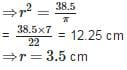∴ Circumference of the circle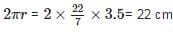Test: Area Related to Circles - 3 - Question 2

### The area of the square that can be inscribed in a circle of radius 12 cm is

Detailed Solution for Test: Area Related to Circles - 3 - Question 2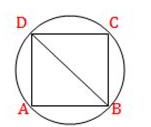According to question, Diameter (BD) = 2 x radius = 2 x 12 = 24 cm
∴ Area of square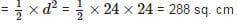Test: Area Related to Circles - 3 - Question 3

### If the area of a circle is equal to the area of a square, then the ratio of their perimeters is

Detailed Solution for Test: Area Related to Circles - 3 - Question 3

Let the radius of the circle be rr and the side of the square be a.a. Then according to the question,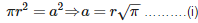Now, Ratio of their perimeters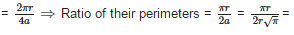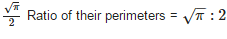Test: Area Related to Circles - 3 - Question 4

A piece of wire 20cm long is bent into the form of an arc of a circle subtending an angle of 60 at its centre. The radius of the circle is

Detailed Solution for Test: Area Related to Circles - 3 - Question 4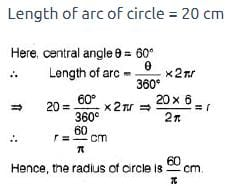Test: Area Related to Circles - 3 - Question 5

The area of a sector of a circle with radius 21cm and sector angle 120 is

Detailed Solution for Test: Area Related to Circles - 3 - Question 5

Area of the sector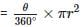⇒ Area of the sector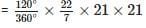= 462 sq. cm

Test: Area Related to Circles - 3 - Question 6

The circumference of a circle whose diameter is 4.2cm is

Detailed Solution for Test: Area Related to Circles - 3 - Question 6

Given : Diameter (d) = 4.2 cm
∴ Circumference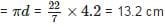Test: Area Related to Circles - 3 - Question 7

The area of the circle that can be inscribed in a square of side 10cm is

Detailed Solution for Test: Area Related to Circles - 3 - Question 7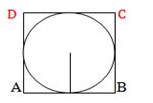Since if a circle inscribed a square. then the radius of the circle is half of to side of square.
∴  Radius = 10/2 = 5 cm
∴ Area of the circle = πr2 = π(5)2 = 25π sq. cm

Test: Area Related to Circles - 3 - Question 8

The area of a square that can be inscribed in a circle of radius 10cm is

Detailed Solution for Test: Area Related to Circles - 3 - Question 8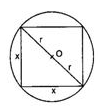Given: Radius (r) = 10 cm
Let side of the square be x cm
Now. using Pythagoras theorem.
x+ x2 = (2r)⇒ 2x2 = (20)2
⇒ 2x2 = 400 ⇒ x2 = 200 sq. cm
Therefore. area of the square = 200 sq. cm.

Test: Area Related to Circles - 3 - Question 9

A square of side 7cmis inscribed in a circle. The area enclosed between the circle and the square is

Detailed Solution for Test: Area Related to Circles - 3 - Question 9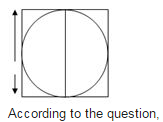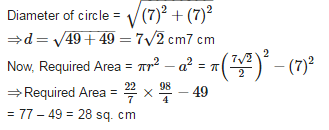Test: Area Related to Circles - 3 - Question 10

The angle described b the minute hand between 4.00 pm and 4.25 pm is

Detailed Solution for Test: Area Related to Circles - 3 - Question 10

Time duration between 4.00 om and 4.25 pm = 25 minutes
∵ Angle described by minute hand in 60 minutes = 360°
∴ Angle described by minute hand in 25 minutes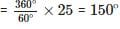Test: Area Related to Circles - 3 - Question 11

The length of the wire is 66m. The number of circles of circumference 13.2cm can be made from the wire is

Detailed Solution for Test: Area Related to Circles - 3 - Question 11

Given: Length of wire = 66 m = 6600 cm and Circumference = 13.2 cm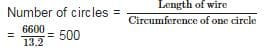Test: Area Related to Circles - 3 - Question 12

If the perimeter of a circle is equal to that of a square, then the ratio of their areas is

Detailed Solution for Test: Area Related to Circles - 3 - Question 12

Let the radius of the circle be rr and side of the square be a. Then, according to question,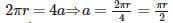Now, ratio of their areas,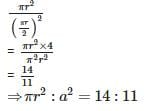Test: Area Related to Circles - 3 - Question 13

If the area and circumference of a circle are numerically equal, then its radius is

Detailed Solution for Test: Area Related to Circles - 3 - Question 13

Let radius of the circle berunits.
∴ Area of the circle = Circumference of the circle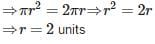Test: Area Related to Circles - 3 - Question 14

On a square handkerchief, nine circular designs each of radius 7cmare made. The area of the remaining portion of the handkerchief is

Detailed Solution for Test: Area Related to Circles - 3 - Question 14

Here Side of square ABCD = AB = 3 x diameter of circular design
⇒ AB = 3 x (2 x 7) = 42 cm
∴ Area of square = 42 x 42 = 1764 cm2
And Area of one circular design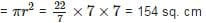And Area of one circular design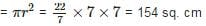∴ Area of 9 circular designs = 154 x 9 = 1386 sq. cm
∴ Area of remaining portion of handkerchief = 1764 - 1386 = 378 sq. cm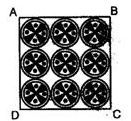Test: Area Related to Circles - 3 - Question 15

A horse is tied to a peg at one corner of a square shaped gross field of side 25m by means of a 14m long rope. The area of that part of the field in which the horse can graze is

Detailed Solution for Test: Area Related to Circles - 3 - Question 15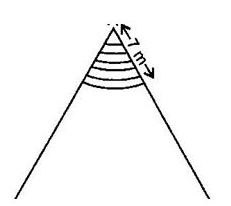Area of the shaded region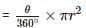Area of the shaded region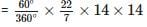Area of the shaded region = 154 sq. cm

Test: Area Related to Circles - 3 - Question 16

The perimeter of a protractor is

Detailed Solution for Test: Area Related to Circles - 3 - Question 16

Let radius of the protractor be r
∴ Perimeter of protractor = Perimeter of semicircle + Diameter of semicircle ⇒ Perimeter of protractor = πr+2r

Test: Area Related to Circles - 3 - Question 17

The radius of a circle whose circumference is equal to the sum of the circumferences of the two circles of diameters 36cm and 20cm is

Detailed Solution for Test: Area Related to Circles - 3 - Question 17

Let required radius be R. Then according to the question,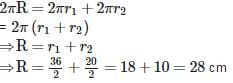Test: Area Related to Circles - 3 - Question 18

If the radius of a circle is increased by 100%, then its area is increased by

Detailed Solution for Test: Area Related to Circles - 3 - Question 18

Area of the circle with radius r =πr2
Now, New Radius = r + 100% of r = r + r = 2r
∴ New Area = π(2r)2 - 4πr2
∴ Increased Area = 4πr2 -  πr2 = 3πr2
And Area increased in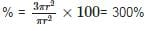Test: Area Related to Circles - 3 - Question 19

If the area of a circle is ‘A’, radius of the circle is ‘r’ and its circumference is ‘C’, then

Detailed Solution for Test: Area Related to Circles - 3 - Question 19

Here, Area of circle (A) = πr2 and Circumference of circle (C) = 2πr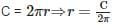Putting value of C in A = πr2, we get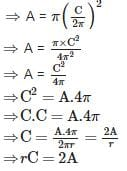Test: Area Related to Circles - 3 - Question 20

A light house throws light forming sector of radius 21 m with central angle 120. The area covered by it is

Detailed Solution for Test: Area Related to Circles - 3 - Question 20

Area of the sector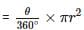⇒ Area of the sector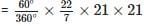⇒ Area of the shaded region = 462 sq. cm

Test: Area Related to Circles - 3 - Question 21

The circumference of a circle exceeds its diameter by 120cm, then its radius is

Detailed Solution for Test: Area Related to Circles - 3 - Question 21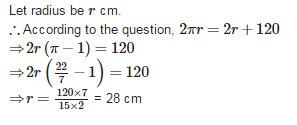Test: Area Related to Circles - 3 - Question 22

If the circumference of a circle and the perimeter of a square are equal, then

Detailed Solution for Test: Area Related to Circles - 3 - Question 22

Let the radius of the circle be r and side of the square be a.
Then, according to question,
2πr = 4a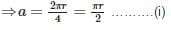Now, ratio of their areas,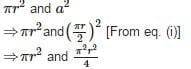Therefore, Area of the circle > Area of the square

Test: Area Related to Circles - 3 - Question 23

The distance around the circle is called its

Detailed Solution for Test: Area Related to Circles - 3 - Question 23

The distance around the circle is called its circumference

Test: Area Related to Circles - 3 - Question 24

The area of a quadrant of a circle whose circumference is 88cm is

Detailed Solution for Test: Area Related to Circles - 3 - Question 24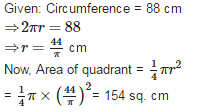Test: Area Related to Circles - 3 - Question 25

If a chord of a circle of radius14cm subtends a right angle at the centre of the circle, then the area of the sector is

Detailed Solution for Test: Area Related to Circles - 3 - Question 25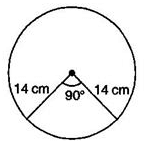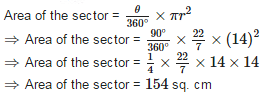## Mathematics (Maths) Class 10

53 videos|369 docs|138 tests
 Use Code STAYHOME200 and get INR 200 additional OFF Use Coupon Code
Information about Test: Area Related to Circles - 3 Page
In this test you can find the Exam questions for Test: Area Related to Circles - 3 solved & explained in the simplest way possible. Besides giving Questions and answers for Test: Area Related to Circles - 3, EduRev gives you an ample number of Online tests for practice

## Mathematics (Maths) Class 10

53 videos|369 docs|138 tests